# Part 2: Understanding the NVL/COALESCE Expression

Now, on to the second part of our journey with the NVL/Coalesce Expression!

Coalesce Expression & Return Types

When a Coalesce/NVL expression is applied, all inputs/arguments should be the same datatype, as the Coalesce function will return the first non-null value of that datatype. If the arguments are different data types such as strings and integers, then Periscope will return an error message.

This example returns a string (indicated by the “Ab” symbol next to the Coalesce” found in the Statistics Tab):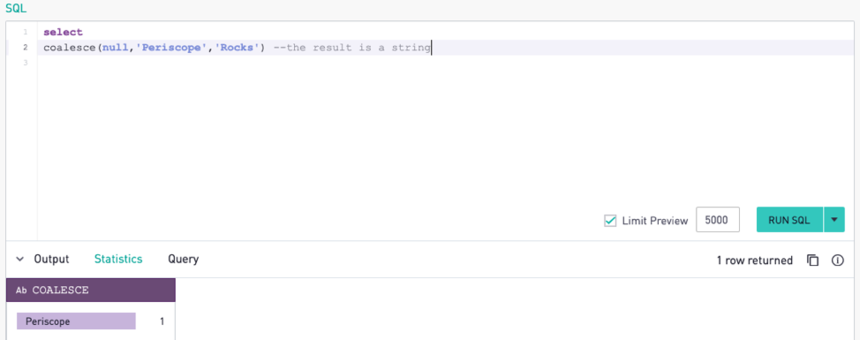This example has more than one data type, and we get an error: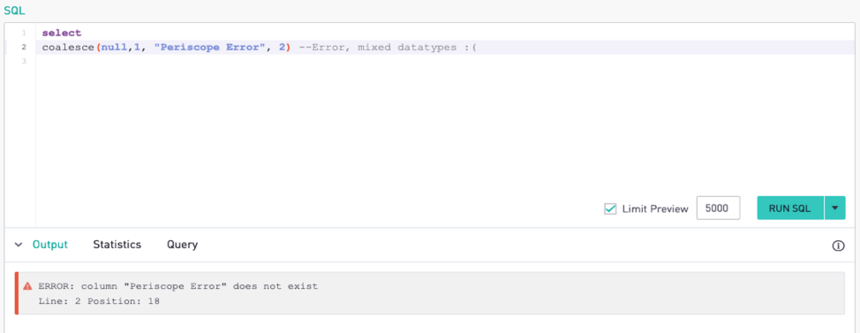Nulls in Columns

Now, let’s apply our knowledge of the coalesce expression to this dataset of local inspection scores of San Francisco restaurants. In the columns selected, we can see that there are null values for some of the entries.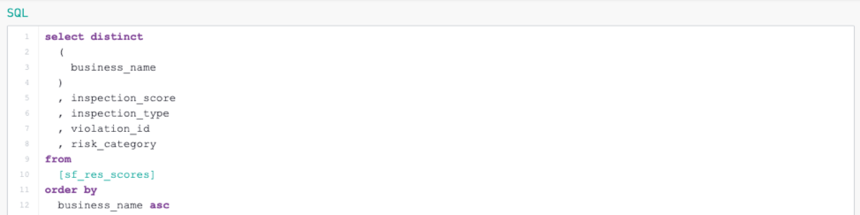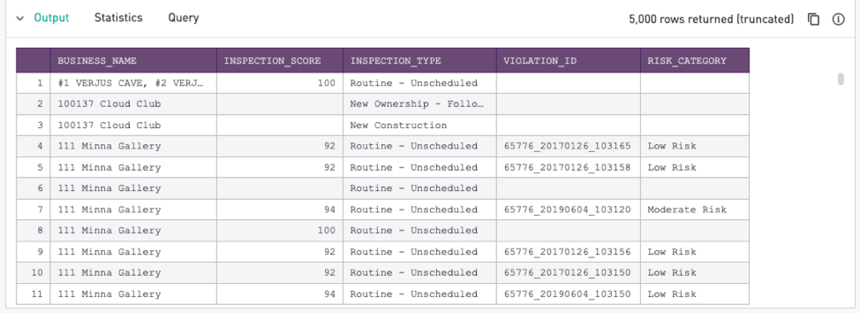If we wanted to clean up or replace the nulls in this dataset, we would first check the data types (the data types can be found in the sidebar such as the inspection score is noted with # as an integer).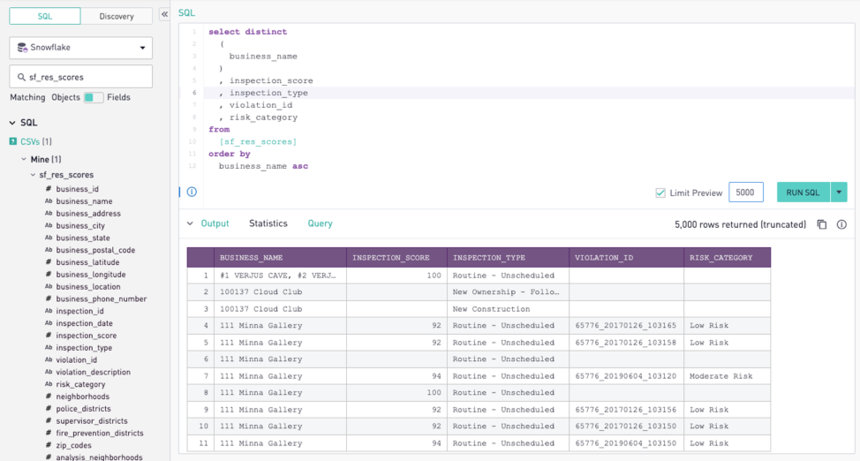As mentioned earlier, if the field’s data type is an integer, then the return value for the null would also have to be an integer, and the same logic would apply for strings, booleans, and e.t.c. For example, the format for integers would be coalesce(column_name, 0) or coalesce(column_name, ‘none’) for strings.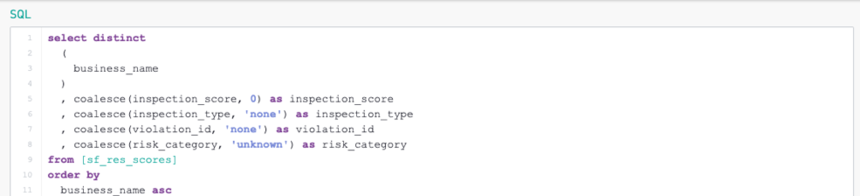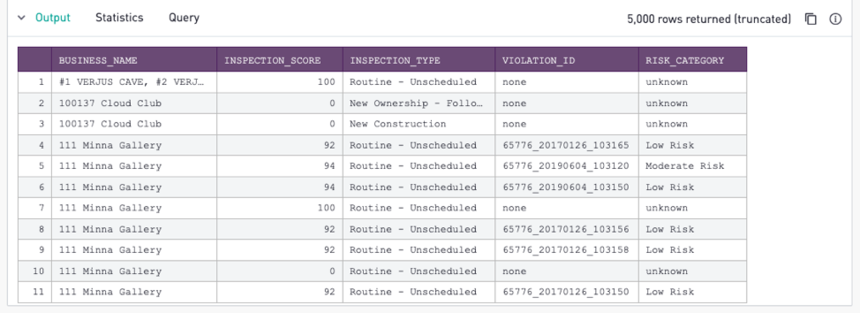Now, what if we had columns with null values involved in a calculation? In this example, let’s say that we wanted to calculate the average of the critical reading, mathematics, and writing test scores for a few schools. In the case of null values for each of these columns, we want to replace them with zeros. It should be noted that for most statistical analyses of averages, replacing the null values with zeros wouldn’t necessarily be representative of the entire dataset, and therefore can be misleading.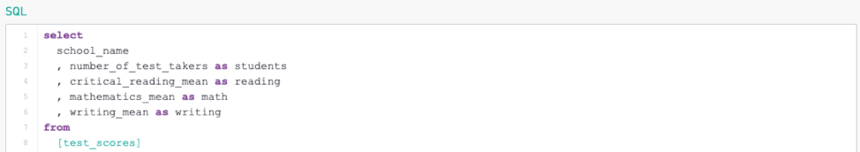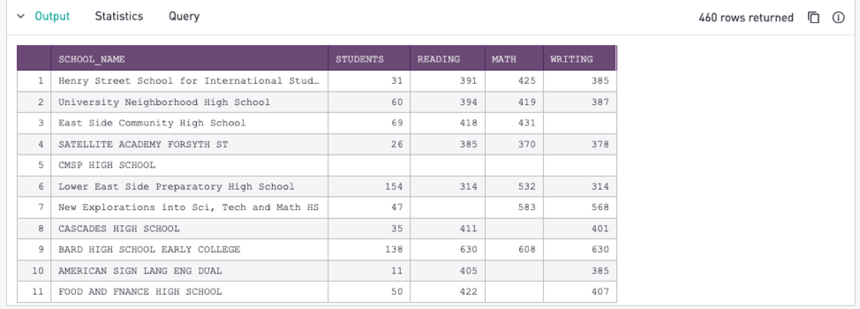First, let’s set all the null values to zeros.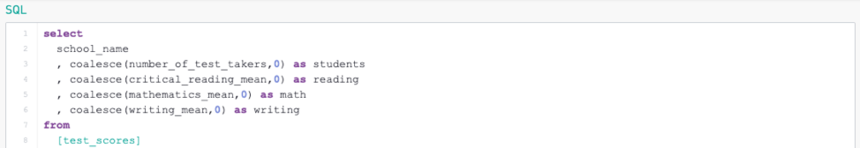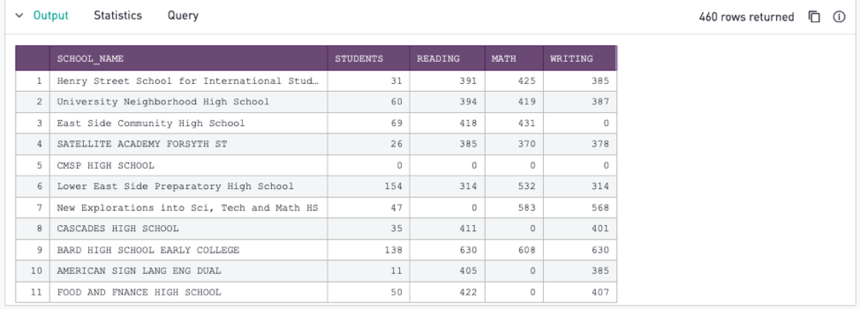Then we can add up all the reading, mathematics, and writing test scores as the “overall_score.”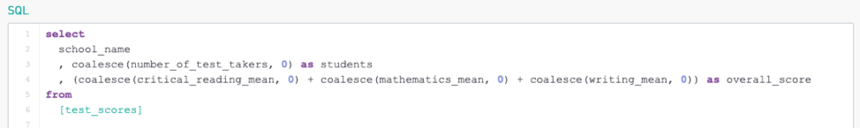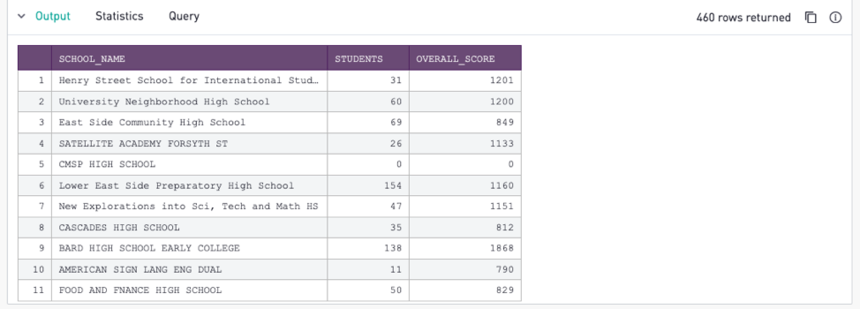Finally, let’s divide the “overall_score” by the number of testing categories (three), and set that result as the “overall_average.”

Periscope Tip: It should be noted that if we wanted to divide by the values in a column where there are nulls, the lowest return value for the coalesce expression would be ‘1’--coalesce (column_name,1). As in the case of division, we can’t divide by zero.

Ta-da! We have the overall average of the three test scores for each school!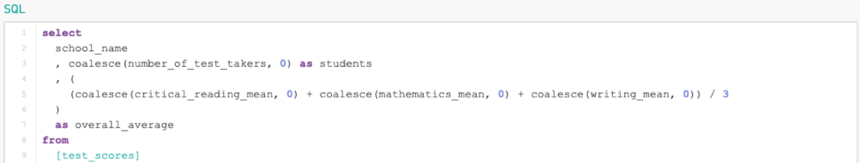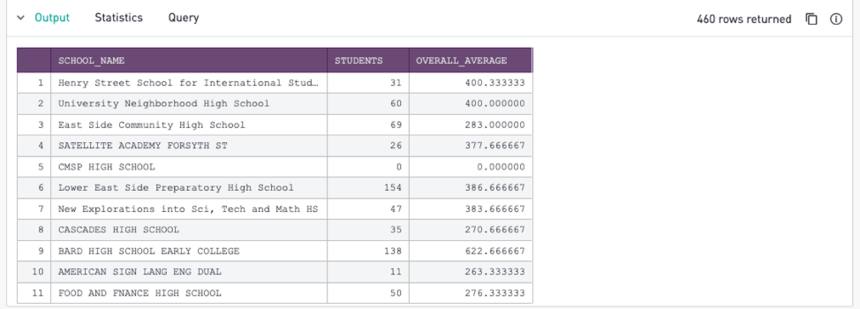Final Periscope Tip: If you wanted to use the avg() function on a column with null values. Then we would still want to replace the null values with zeros, because despite the avg() function ignoring null values—the aggregate function still counts their occurrence in the divisor. For example, in the case using avg() on a column with the values of (10,10,10, NULL), that calculation actually translates as (10+10+10)/3 which returns 10, instead of (10+10+10+0)/4 with the actual average of 7.5. By adding a Coalesce/NVL expression with the average function such as avg(coalesce(column_name,0)), we can effectively solve this issue.